## Prim’s and Kruskal’s Algorithms-

Before you go through this article, make sure that you have gone through the previous articles on Prim’s Algorithm & Kruskal’s Algorithm.

We have discussed-

• Prim’s and Kruskal’s Algorithm are the famous greedy algorithms.
• They are used for finding the Minimum Spanning Tree (MST) of a given graph.
• To apply these algorithms, the given graph must be weighted, connected and undirected.

Some important concepts based on them are-

## Concept-01:

If all the edge weights are distinct, then both the algorithms are guaranteed to find the same MST.

### Example-

Consider the following example-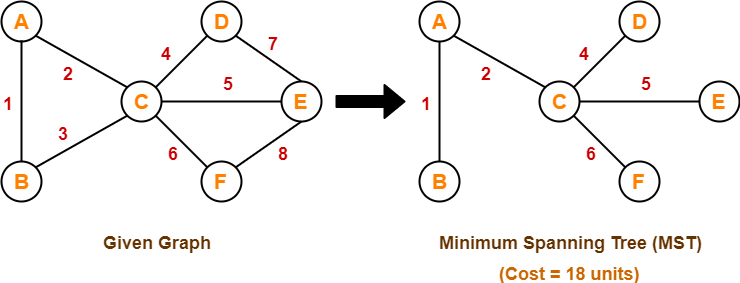Here, both the algorithms on the above given graph produces the same MST as shown.

## Concept-02:

• If all the edge weights are not distinct, then both the algorithms may not always produce the same MST.
• However, cost of both the MSTs would always be same in both the cases.

### Example-

Consider the following example-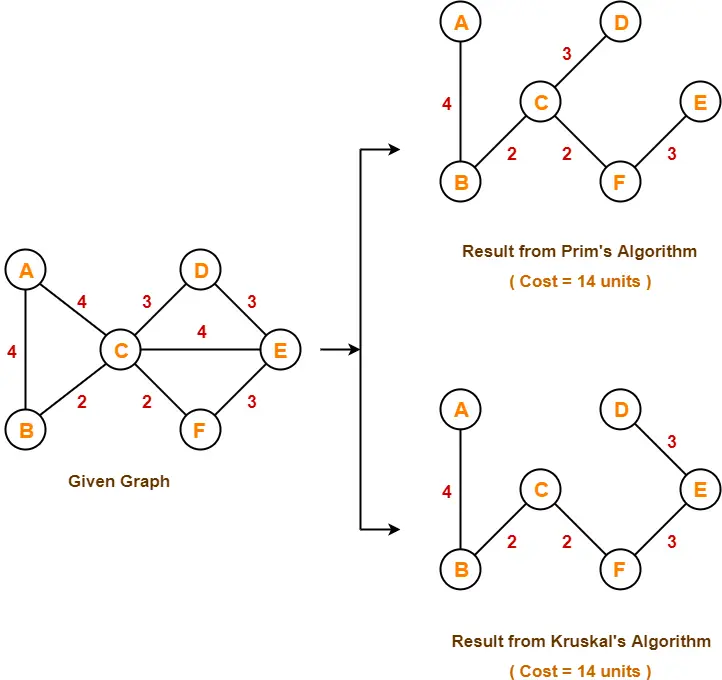Here, both the algorithms on the above given graph produces different MSTs as shown but the cost is same in both the cases.

## Concept-03:

Kruskal’s Algorithm is preferred when-

• The graph is sparse.
• There are less number of edges in the graph like E = O(V)
• The edges are already sorted or can be sorted in linear time.

Prim’s Algorithm is preferred when-

• The graph is dense.
• There are large number of edges in the graph like E = O(V2).

## Concept-04:

Difference between Prim’s Algorithm and Kruskal’s Algorithm-

 Prim’s Algorithm Kruskal’s Algorithm The tree that we are making or growing always remains connected. The tree that we are making or growing usually remains disconnected. Prim’s Algorithm grows a solution from a random vertex by adding the next cheapest vertex to the existing tree. Kruskal’s Algorithm grows a solution from the cheapest edge by adding the next cheapest edge to the existing tree / forest. Prim’s Algorithm is faster for dense graphs. Kruskal’s Algorithm is faster for sparse graphs.

To gain better understanding about Difference between Prim’s and Kruskal’s Algorithm,

Watch this Video Lecture

Next Article- Linear Search

Get more notes and other study material of Design and Analysis of Algorithms.

Watch video lectures by visiting our YouTube channel LearnVidFun.

## Prim’s Algorithm-

• Prim’s Algorithm is a famous greedy algorithm.
• It is used for finding the Minimum Spanning Tree (MST) of a given graph.
• To apply Prim’s algorithm, the given graph must be weighted, connected and undirected.

## Prim’s Algorithm Implementation-

The implementation of Prim’s Algorithm is explained in the following steps-

### Step-01:

• Randomly choose any vertex.
• The vertex connecting to the edge having least weight is usually selected.

### Step-02:

• Find all the edges that connect the tree to new vertices.
• Find the least weight edge among those edges and include it in the existing tree.
• If including that edge creates a cycle, then reject that edge and look for the next least weight edge.

### Step-03:

• Keep repeating step-02 until all the vertices are included and Minimum Spanning Tree (MST) is obtained.

## Prim’s Algorithm Time Complexity-

Worst case time complexity of Prim’s Algorithm is-

• O(ElogV) using binary heap
• O(E + VlogV) using Fibonacci heap

### Time Complexity Analysis

• If adjacency list is used to represent the graph, then using breadth first search, all the vertices can be traversed in O(V + E) time.
• We traverse all the vertices of graph using breadth first search and use a min heap for storing the vertices not yet included in the MST.
• To get the minimum weight edge, we use min heap as a priority queue.
• Min heap operations like extracting minimum element and decreasing key value takes O(logV) time.

So, overall time complexity

= O(E + V) x O(logV)

= O((E + V)logV)

= O(ElogV)

This time complexity can be improved and reduced to O(E + VlogV) using Fibonacci heap.

## Problem-01:

Construct the minimum spanning tree (MST) for the given graph using Prim’s Algorithm-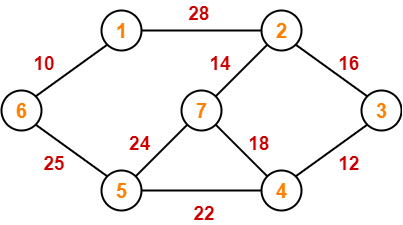## Solution-

The above discussed steps are followed to find the minimum cost spanning tree using Prim’s Algorithm-

### Step-01: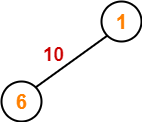### Step-02: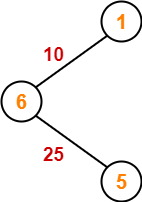### Step-03: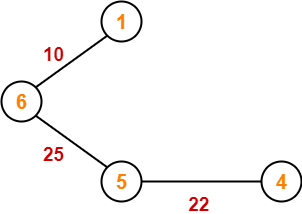### Step-04: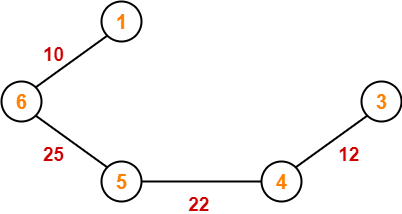### Step-05: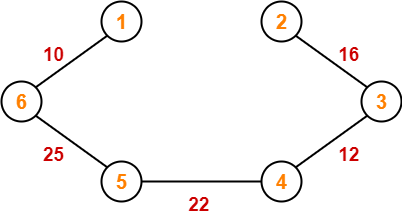### Step-06: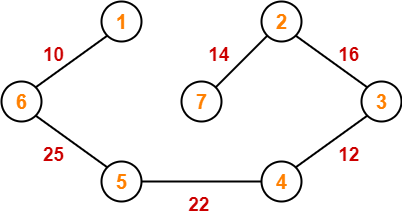Since all the vertices have been included in the MST, so we stop.

Now, Cost of Minimum Spanning Tree

= Sum of all edge weights

= 10 + 25 + 22 + 12 + 16 + 14

= 99 units

## Problem-02:

Using Prim’s Algorithm, find the cost of minimum spanning tree (MST) of the given graph-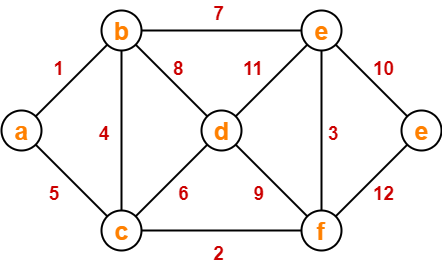## Solution-

The minimum spanning tree obtained by the application of Prim’s Algorithm on the given graph is as shown below-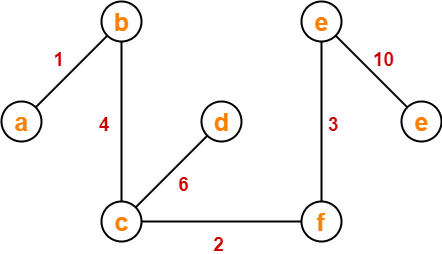Now, Cost of Minimum Spanning Tree

= Sum of all edge weights

= 1 + 4 + 2 + 6 + 3 + 10

= 26 units

To gain better understanding about Prim’s Algorithm,

Watch this Video Lecture

To practice previous years GATE problems based on Prim’s Algorithm,

Watch this Video Lecture

Next Article- Kruskal’s Algorithm

Get more notes and other study material of Design and Analysis of Algorithms.

Watch video lectures by visiting our YouTube channel LearnVidFun.